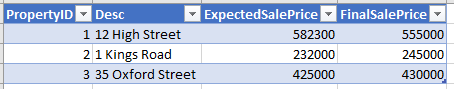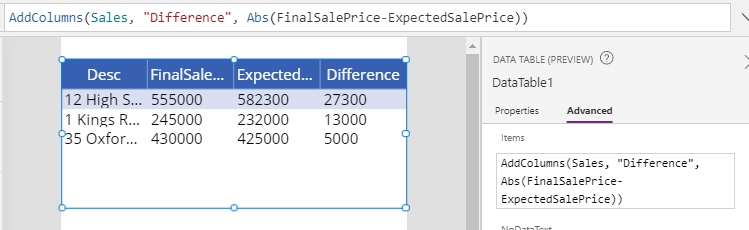# Data - How to check if a value falls within a certain range or tolerance

When performing calculations, it can be very useful to determine if an input value falls within a certain range tolerance level. This post describes one way to carry out this task.

In cases where we want determine if an input value falls within a certain tolerance level, we can enlist the help of the Abs function. This function returns the absolute value of a number. In other words, it returns the non-negative value of the input value that we pass to the function. The documentation for this function is here.

https://docs.microsoft.com/en-gb/powerapps/maker/canvas-apps/functions/function-numericals

## Example of how to call the Abs function

As an example, let's take the following table of property details. This data contains the expected and actual sales prices of properties.

The actual sales prices maybe more with less than the expected sales price, and the example requirement is to determine whether the actual sales price falls within 10% of the expected value.We can display the absolute difference between these two values by adding a gallery or data table control to a screen, and setting the Items property to the following formula:

`AddColumns(Sales,            "Difference",            Abs(FinalSalePrice-ExpectedSalePrice))`
As the following screenshot shows, the data table control correctly displays the difference between the two values.## How to calculate the variance as a percentage

As a logical extension of this formula, we can now add an extra column that displays the percentage variance from the expected sales price:

`AddColumns(Sales,            "Difference",            Abs(FinalSalePrice-ExpectedSalePrice),           "PercentageDiff",           (Abs(FinalSalePrice-ExpectedSalePrice)/ExpectedSalePrice)*100 )`
The screenshot beneath illustrates this output.## Conclusion

To determine if values falls within a certain tolerance level, we can call the Abs function. This post demonstrated how we can apply this function to table or collection of data.
•   Categories:
• sums
Related posts